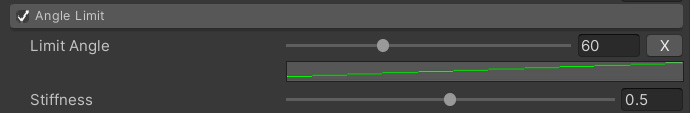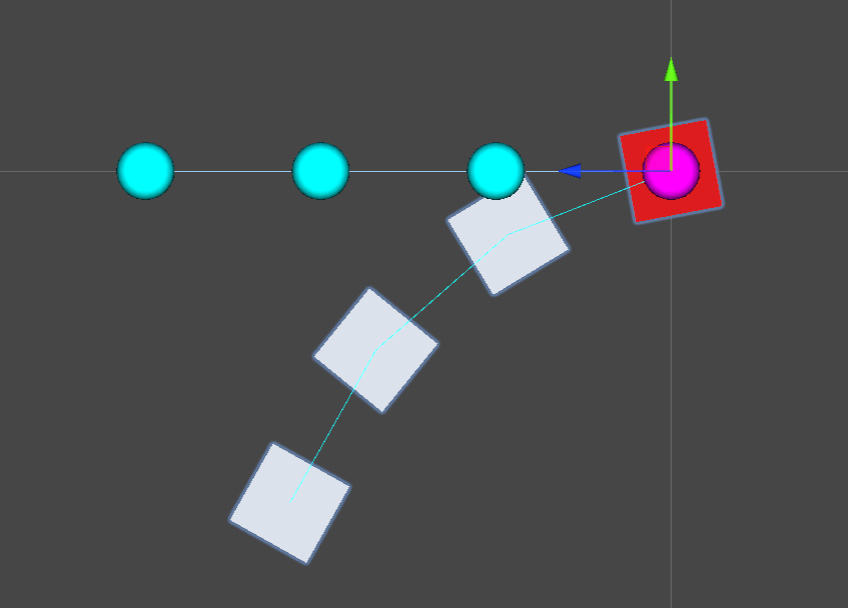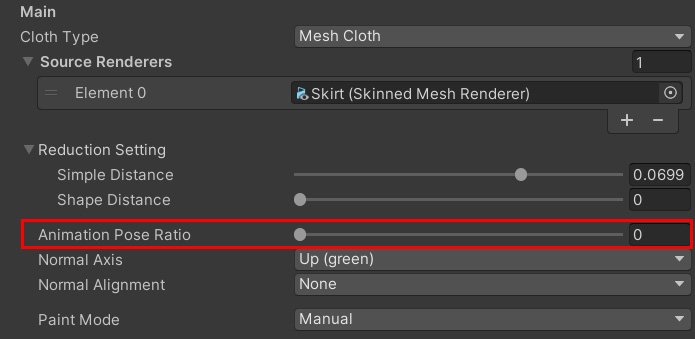# Angle Limit

#### Angle limitLimits the angle at which edges can bend relative to the baseline.

 Limit Angle The angle (Deg) that each edge of the baseline can bend from its parent.By using a curve, you can adjust according to the depth of the vertices. Stiffness repulsive force.Lowering the value will make it return softly at the limit.

For example, if the limit angle of each vertex is uniformly set to 20 degrees, it will be as follows.
The light blue ball and line are the original posture.#### Effect of Animation Posture Ratio

Shape restoration is affected by the animation pose ratio.
This is the ratio of whether the restoration reference should be the initial pose or the current animation pose.